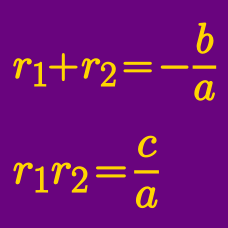Algebra

# Vieta's Formula

#### Challenge Quizzes

What is the product of roots of the quadratic equation

$2 x^2 - 6 x + 36 = 0,$

If the two roots of the quadratic equation $x^2-12x-2=0$ are $\alpha$ and $\beta$, what is $\alpha^2+\beta^2$?

The difference between the two roots of the quadratic equation $x^2+Ax+B=0$ is $12$ and the larger root is $3$ times the smaller root. What is the value of $A+B$?

What are the sum of roots of the quadratic equation

$x^2 - 9 x + 15 = 0?$

Let $\alpha$ and $\beta$ be the two roots of the quadratic equation $x^2-16x+63=0.$ What is the value of $\alpha+\beta+\alpha\beta$?

×